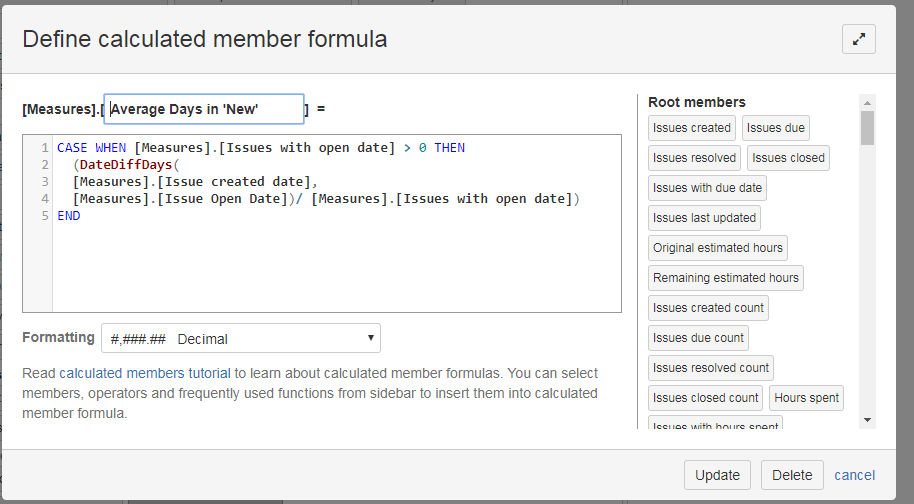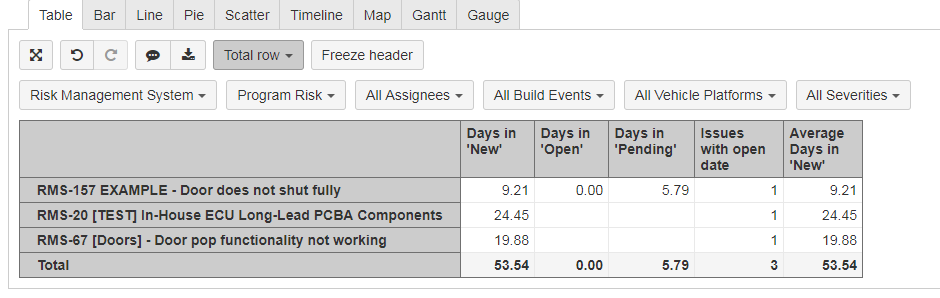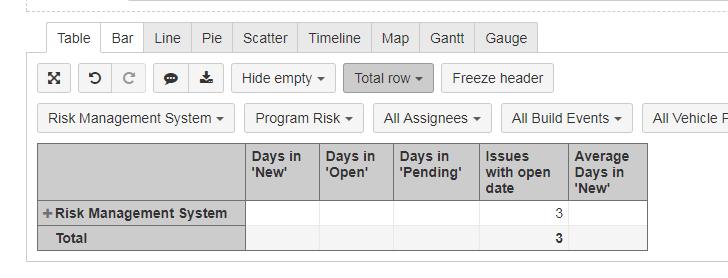cancel
Showing results for
Did you mean:
See all
See all
##### Top groups
Explore all groups

# It's not the same without you

Join the community to find out what other Atlassian users are discussing, debating and creating.# Calculate Total Number of days between two date stamps for multiple issues (EazyBi)

Essentially I need to find the aggregate of "Days in 'New'" across issues.

I have a table that will spit out the total but I need a formula that includes the total number of "Days in 'New'" and divide it by the issues with open date to get an average.

So far I have only been able to calculate the "Days in New" for each individual issue.

I am using two different date stamps to calculate days in new/open/pending.

This was my first attempt at the formula:And these are the results. As you can see, If I sort by individual issues it works fine but when I collapse it to get an average of the project I don't get any results.See above

## 1 comment

Hi Simon

You are creating a calculation using Issue properties "Issue created date", "Issue open date". Issue properties work at issue level only. Therefore a calculation has a result for individual issues but not for total calculation.

For total calculation, you would like to define a set of issues and calculate an average over this set.

AVG(set, numeric_expression)

Set should be an issue set filtered by this date

Numeric_expression is the one you would like to use for average calculation - date diff days between issue creation date and an open date.

`NonZero(AVG( Filter( Descendants([Issue].CurrentMember, [Issue].[Issue]), NOT isempty([Measures].[Issue open date]) AND DateInPeriod( [Measures].[Issue open date], [Time].CurrentHierarchyMember ) AND ([Measures].[Issues created], [Time].CurrentHierarchy.DefaultMember) > 0), DateDiffDays ([Measures].[Issue created date], [Measures].[Issue open date])))`

Please set the formatting of your calculated measure to decimal as well.

Daina / support@eazybi.com